Courses

# Gaseous Introduction (Part - 2) Chemistry Notes | EduRev

## Physical Chemistry

Created by: Asf Institute

## Chemistry : Gaseous Introduction (Part - 2) Chemistry Notes | EduRev

The document Gaseous Introduction (Part - 2) Chemistry Notes | EduRev is a part of the Chemistry Course Physical Chemistry.
All you need of Chemistry at this link: Chemistry

6. Brownian motion

Brownian motion is the mathematical model used to describe the random movement of particles suspended in a fluid. The gas particle animation, using pink and green particles, illustrates how this behavior results in the spreading out of gases (entropy). These events are also described by particle theory.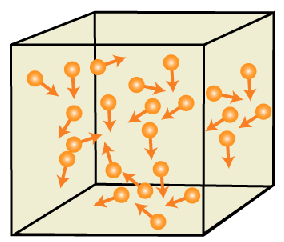Brownian motion

Since it is at the limit of (or beyond) current technology to observe individual gas particles (atoms or molecules), only theoretical calculations give suggestions about how they move, but their motion is different from Brownian motion because Brownian motion involves a smooth drag due to the frictional force of many gas molecules, punctuated by violent collisions of an individual (or several) gas molecule(s) with the particle.

The particle (generally consisting of millions or billions of atoms) thus moves in a jagged course, yet not so jagged as would be expected if an individual gas molecule were examined.

7. Intermolecular forces

As discussed earlier, momentary attractions (or repulsions) between particles have an effect on gas dynamics. In physical chemistry, the name given to these intermolecular forces is vander Waals force.

These forces play a key role in determining physical properties of a gas such as viscosity and flow rate (see physical characteristics section). Ignoring these forces in certain conditions allows a real gas to be treated like an ideal gas. This assumption allows the use of ideal gas laws which greatly simplifies calculations.

Proper use of these gas relationships requires the kinetic-molecular theory (KMT). When gas particles experience intermolecular forces they gradually influence one another as the spacing between them is reduced (the hydrogen bond model illustrates one example).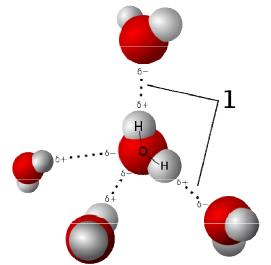Inter-molecule forces between H2O molecule

In the absence of any charge, at some point when the spacing between gas particles is greatly reduced they can no longer avoid collisions between themselves at normal gas temperatures.

Another case for increased collisions among gas particles would include a fixed volume of gas, which upon heating would contain very fast particles. This means that these ideal equations provide reasonable results except for extremely high pressure (compressible) or high temperature (ionized) conditions. All of these excepted conditions allow energy transfer to take place within the gas system. The absence of these internal transfers is what is referred to as ideal conditions in which the energy exchange occurs only at the boundaries of the system. Real gases experience some of these collisions and intermolecular forces. When these collisions are statistically negligible (incompressible), results from these ideal equations are still meaningful. If the gas particles are compressed into close proximity they behave more like a liquid (read fluid dynamics).

8. Ideal Gases

These gases obey gas laws like Boyle’s law, Charle’s law, Avogadro’s law at all temperature and pressures. There are no intermolecular forces of attractions and no change in their energy even if their volume increases. But in reality, existence of ideal gases is only hypothesis.

The ideal gas law does not make an assumption about the specific heat of a gas. In the most general case, the specific heat is a function of both temperature and pressure. If the pressure-dependence is neglected (and possibly the temperature-dependence as well) in a particular application, sometimes the gas is said to be a perfect gas.

For an ideal gas, the ideal gas law applies without restrictions on the specific heat. An ideal gas is a simplified "real gas" with the assumption that the co mpressibility factor Z is set to 1 meaning that this pneumatic ratio remains constant. A compressibility factor of one also requires the four state variables to follow the ideal gas law.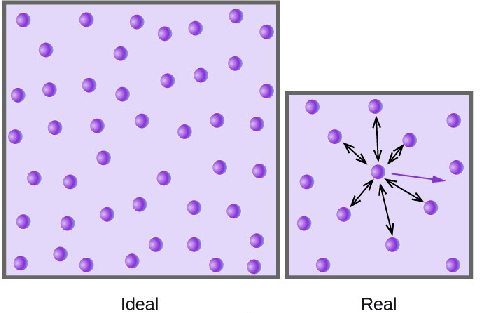Intermolecular forces between ideal and real gases

9. Real Gases

Each one of the assumptions listed below adds to the complexity of the problem's solution. As the density of a gas increases with rising pressure, the intermolecular forces play a more substantial role in gas behavior which results in the ideal gas law no longer providing "reasonable" results. At the upper end of the temperature ranges (say 1300K), the complex fuel particles absorb internal energy by means of rotations and vibrations that cause their specific heats to vary from those of diatomic molecules and noble gases. At more than double that temperature, electronic excitation and dissociation of the gas particles begins to occur causing the pressure to adjust to a greater number of particles (transition from gas to plasma). Finally, all of the thermodynamic processes were presumed to describe uniform gases whose velocities varied according to a fixed distribution. Using a non-equilibrium situation implies the flow field must be characterized in some manner to enable a solution. One of the first attempts to expand the boundaries of the ideal gas law was to include coverage for different thermodynamic processes by adjust ing the equation to read pVn = constant and then varying then through different values such as the specific heat ratio, γ.

These gases obey gas laws at very high pressure and very low temperatures and at –273°C, their volume do not reduce to zero, are known as real gases. Considerable forces of attraction & appreciable size of molecules. These under " certain conditions" behalve like ideal.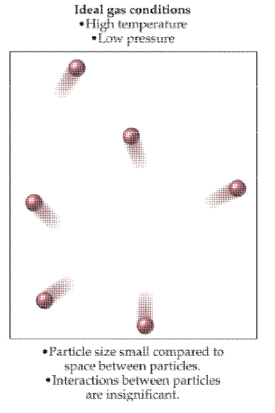Ideal gas condition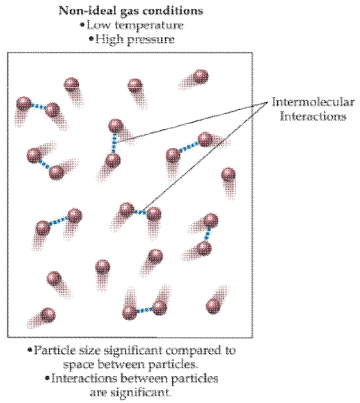Non-ideal gas condition

Real gas effects include those adjustments made to account for a greater range of gas behavior:

· Compressibility effects (Z allowed to vary from 1.0)

· Variable heat capacity (specific heats vary with temperature)

· Vander Waals forces (related to compressibility, can substitute other equations of state)

10. Experimental derived gas laws: Relationship between various parameter of the gas. Gaseous state is the only state that allows a quantitative description between the four parameters, P, V, T & n. The relationship which connects the four variables is known as equation of state, which can be obtained experimentally from the following gas laws.

1. Boyle’s law (Robert Boyle, 1662)

Boyle's Law was perhaps the first expression of an equation of state. In 1662 Robert Boyle performed a series of experiments employing a J-shaped glass tube, which was sealed on one end. Mercury was added to the tube, trapping a fixed quantity of air in the short, sealed end of the tube. Then the volume of gas was carefully measured as additional mercury was added to the tube. The pressure of the gas could be determined by the difference between the mercury level in the short end of the tube and that in the long, open end. The image of Boyle's Equipment shows some of the exotic tools used by Boyle during his study of gases.

Through these experiments, Boyle noted that the pressure exerted by a gas held at a constant temperature varies inversely with the volume of the gas. For example, if the volume is halved, the pressure is doubled; and if the volume is doubled, the pressure is halved. Given the inverse relationship between pressure and volume, the product of pressure (P) and volume (V) is a constant (k) for a given mass of confined gas as long as the temperature is constant. Stated as a formula, thus is:

P ∝ 1/V

or PV = constant (K) or P1V1 = P2V2.

Graphical Analysis: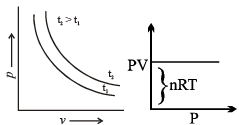2. Charle’s law (Jacques Charle’s 1987)

In 1787, the French physicist and balloon pioneer, Jacques Charles, found that oxygen, nitrogen, hydrogen, carbon dioxide, and air expand to the same extent over the same 80 kelvin interval. He noted that, for an ideal gas at constant pressure, the volume is directly proportional to its temperature:

The volume of a given mass of a gas at constant pressure increase or decreases by 1/273 of its volume of 0°C for each degree rise or fall of temperature.

· The volume of a fixed mass of the gas at constant pressure is directly proportional to its temperature in Kelvin.

· Mathematical representation: At constant pressure (P) and fixed number of moles (n).

V ∝ T or V/T = constant (K)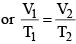The value of K depends upon mass, pressure and nature of gas.

Graphical Analysis:

The law can be represented by plotting V vs T at constant pressure.  Such plot is called isobar.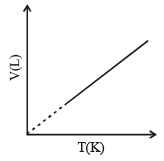The volume of the gas is a linear function of the temperature.

Vt = a + bt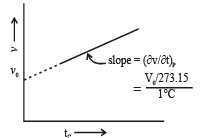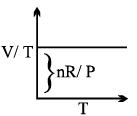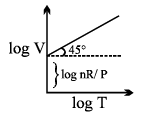3. Gay-Lussac’s Law

In 1802, Joseph Louis Gay-Lussac published results of similar, though more extensive experiments. GayLussac credited Charles' earlier work by naming the law in his honor. Gay-Lussac himself is credited with the law describing pressure, which he found in 1809. It states that the pressure exerted on a container's sides by an ideal gas is proportional to its temperature.

Graphical Analysis: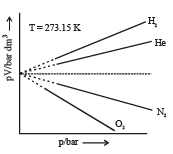According to Avogadro’s law, equal number of molecules of different gases under identical conditions of temperature and pressure occupy the same volume.

Whether the molecules are heavy (e.g. Br2) or light (e.g. H2) gases with equal number of molecules would occupy the same volume.  This leads to one of the most important features of gases that the distance between molecules is much larger than the actual dimensions of molecules, since otherwise, Avogadro’s law would not have been true.

Graphical Analysis: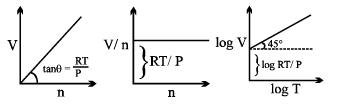11. Universal Gas constant

R = PV/nT

Physical Significance of Gas constant R: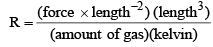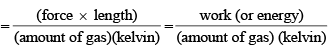Value of Gas Constant in SI units: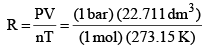= 0.083 14 bar dm3 K–1 mol–1

The gas constant(R) in various units

8.31451 J K-1 mol-1

8.20578 x 10-2 l atm K-1 mol-1

8.31451 x 10-2 L bar K-1 mol-1

8.31451 Pa m3 K-1 mol-1

62.364 L Torr K-1 mol-1

1.98722 cal K-1 mol-1

12. Concepts of Partial Pressure and Partial Volume

According to Dalton’s law of partial pressure, the total pressure of a mixture of gases is equal to the sum of the partial pressure of the constituent gases.

Partial Pressure in a Gaseous Mixture

Let a mixture of gases have the amount n1 of the first gas, n2 of the second gas and so on. Let the corresponding partial pressures be p1, p2, ..... The total pressure is given by

Ptotal = P1 + P2 + ....

If the gases present in the mixture behave ideally, then it is possible to write separately for each gas,

P1V = n1RT       ....(1a)
P2V = n2RT      ...(1b)

Hence (P1 + P2 + ....) V = (n1 + n2 + ....) RT

i.e.    PtotalV = ntotalRT                 ...(2)

where ntotal is the total amount of gases in the mixture.  Dividing eqs (1a) and (1b) by Eq. (2). we get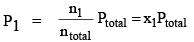..(3a)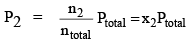...(3b)

13. Graham’s Law of Diffusion & Effusion

The rate of diffusion (or effusion) of a gas is inversely proportional to the square root of its density or molar mass.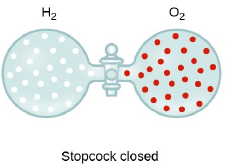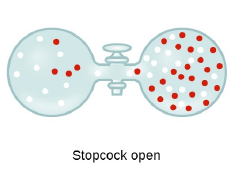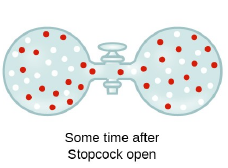Mathematically: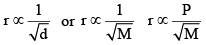[For gases effusing at different pressures]

Where ‘r’ is rate of diffusion of any gas.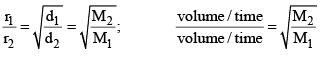d is density at some temperature.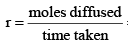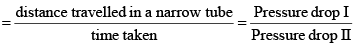Offer running on EduRev: Apply code STAYHOME200 to get INR 200 off on our premium plan EduRev Infinity!

,

,

,

,

,

,

,

,

,

,

,

,

,

,

,

,

,

,

,

,

,

;